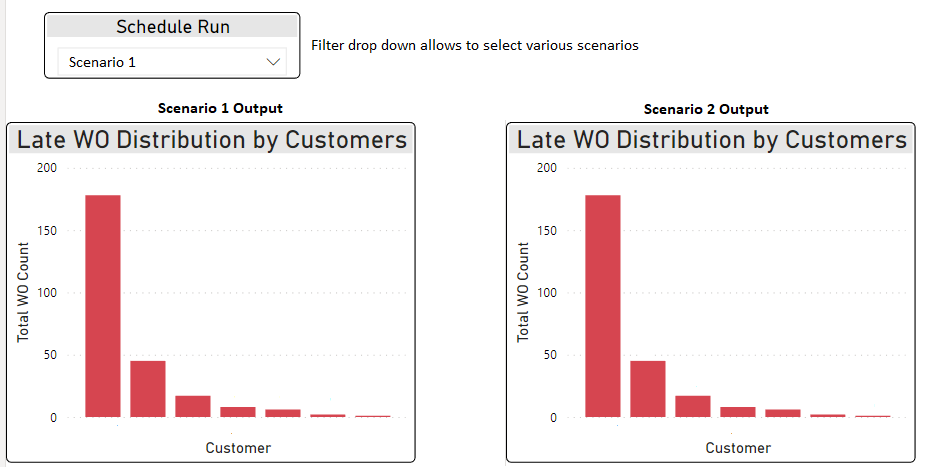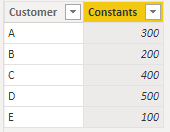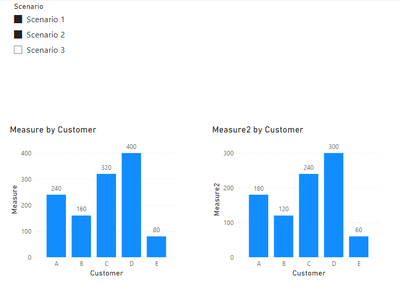cancel
Showing results for
Did you mean:Helper IV

## Linking Multiple Different Bar Charts to Different Selections in filter

Hi Everyone,

I am trying to compare two planning/scheduling scenarios side by side to see which scenario is better. I have a filter from which I can select multiple/two scenarios.

Thanks in advance!!!1 ACCEPTED SOLUTIONCommunity Support

Due to I don't know your data model, here I will show you two workarounds to achieve your goal.

1. If your calculation logic could be producting Constants from Fact table with Constants from Scenario table, you can try this way.``````Measure =
VAR _CONSTANT1 = CALCULATE(SUM(Scenario[Value]),FILTER(Scenario,Scenario[Scenario] = MIN(Scenario[Scenario])))
VAR _CONSTANT2 = CALCULATE(SUM(Sample1[Constants]))
RETURN
_CONSTANT1 * _CONSTANT2``````
``````Measure2 =
VAR _CONSTANT1 = CALCULATE(SUM(Scenario[Value]),FILTER(Scenario,Scenario[Scenario] = MAX(Scenario[Scenario])))
VAR _CONSTANT2 = CALCULATE(SUM(Sample1[Constants]))
RETURN
_CONSTANT1 * _CONSTANT2``````

Result is as below.2. You can create a Fact table with all data from each Scenario.``Measure 2 = CALCULATE(SUM(Sample2[Value]),FILTER(Sample2,Sample2[Scenario] = MIN(Scenario[Scenario])))``
``Measure 3 = CALCULATE(SUM(Sample2[Value]),FILTER(Sample2,Sample2[Scenario] = MAX(Scenario[Scenario])))``

Result is as below.Best Regards,
Rico Zhou

If this post helps, then please consider Accept it as the solution to help the other members find it more quickly.

2 REPLIES 2Community Support

Due to I don't know your data model, here I will show you two workarounds to achieve your goal.

1. If your calculation logic could be producting Constants from Fact table with Constants from Scenario table, you can try this way.``````Measure =
VAR _CONSTANT1 = CALCULATE(SUM(Scenario[Value]),FILTER(Scenario,Scenario[Scenario] = MIN(Scenario[Scenario])))
VAR _CONSTANT2 = CALCULATE(SUM(Sample1[Constants]))
RETURN
_CONSTANT1 * _CONSTANT2``````
``````Measure2 =
VAR _CONSTANT1 = CALCULATE(SUM(Scenario[Value]),FILTER(Scenario,Scenario[Scenario] = MAX(Scenario[Scenario])))
VAR _CONSTANT2 = CALCULATE(SUM(Sample1[Constants]))
RETURN
_CONSTANT1 * _CONSTANT2``````

Result is as below.2. You can create a Fact table with all data from each Scenario.``Measure 2 = CALCULATE(SUM(Sample2[Value]),FILTER(Sample2,Sample2[Scenario] = MIN(Scenario[Scenario])))``
``Measure 3 = CALCULATE(SUM(Sample2[Value]),FILTER(Sample2,Sample2[Scenario] = MAX(Scenario[Scenario])))``

Result is as below.Best Regards,
Rico Zhou

If this post helps, then please consider Accept it as the solution to help the other members find it more quickly.Helper IV

Thank you so much, the 2nd suggestion worked!!!!